Definitions of Square Dance Calls and Concepts
Touch By {fraction} By {fraction} | {anything} [C3A]

Index -->  Plus  |  A1  |  A2  |  C1  |  C2  |  C3A  |  C3B  |  C4  |  NOL  |
Definitions (Text Only) -->  Plus  |  A1  |  A2  |  C1  |  C2  |  C3A  |  C3B  |  C4  |  NOL  |
 Find call:

Touch By fraction By fraction | anything -- [C3A]

 \$B8@8l(BFrom a Single Double Pass Thru, the formation obtained from a Diamond after everyone 1/4 In, or other applicable formations. EN: 10
Single Double Pass Thru, Diamond \$B\$+\$iA40w(B 1/4 In \$B\$7\$?(B formation, \$B\$^\$?\$OB>\$NE,@Z\$J(B formation \$B\$+\$i!%(B JP: 10

Centers Touch the first given fraction and Spread as Others step forward; new Centers Touch the second given fraction or do the anything call. Usually ends in a R-H Diamond or a R-H Two-Faced Line. There are no default fractions for this call. EN: 20
Centers \$B\$,;O\$a\$N(B fraction \$B\$N(B Touch \$B\$r9T\$\$(B Spread \$B\$7\$FB>\$N?M\$OA0?J\$7(B, new Centers \$B\$Ofraction \$B\$N(B Touch \$B\$r9T\$&\$+(B anything \$B\$N%3!<%k\$r9T\$\$\$^\$9!%\$3\$N%3!<%k\$G\$O(B fraction \$B\$,>JN,\$5\$l\$?\$H\$-\$N%G%U%)%k%H\$O\$"\$j\$^\$;\$s!%(B
\$BLuJN,\$5\$l\$?\$H\$-\$K;H\$o\$l\$kCM\$r;X\$98@MU\$G(B,\$B!V(BSquare Thru \$B\$N%G%U%)%k%H\$O(B 4\$B!W\$N\$h\$&\$K;H\$\$\$^\$9!%(B JP: 20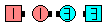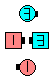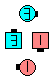Touch By 1/2 By 1/4\$B\$NA0(B Centers Touch 1/2 & Spreadas Others step forward EN: 25 Centers \$B\$,(B Touch 1/2 - Spread, \$BB>\$N?M\$O(B step forward JP: 25 \$B\$N8e(B new Centers Touch 1/4\$B\$N8e(B (\$B=*\$o\$j(B)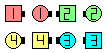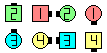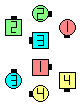Touch By 1/4 By Swing Thru\$B\$NA0(B Centers Touch 1/4 & Spreadas Others step forward EN: 30 Centers \$B\$,(B Touch 1/4 - Spread, \$BB>\$N?M\$O(B step forward JP: 30 \$B\$N8e(B new Centers Swing Thru\$B\$N8e(B (\$B=*\$o\$j(B)

Note: the anything call (which does not have to be a 2-dancer call) is done from Facing dancers. From a Double Pass Thru, consider a Touch By 1/4 By Recycle (it is a facing Recycle). EN: 40
\$BCmanything \$B\$N%3!<%k(B (2 \$B?M\$N%3!<%k\$G\$"\$kI,MW\$O\$"\$j\$^\$;\$s(B) \$B\$O8~\$+\$\$9g\$C\$?\$H\$3\$m\$+\$i9T\$\$\$^\$9!%(BDouble Pass Thru \$B\$+\$i(B, Touch By 1/4 By Recycle (facing Recycle) \$B\$r9M\$(\$F\$_\$F\$/\$@\$5\$\$!%(B JP: 40

\$B1~MQ(B:

• Column of 6 Touch By (3 fractions | anything given); EN: 50
Column of 6 Touch By (3 fractions \$B\$^\$?\$O(B anything \$B\$,M?\$(\$i\$l\$^\$9(B), JP: 50
• Column of 8 Touch By (4 fractions | anything given). EN: 60
Column of 8 Touch By (4 fractions \$B\$^\$?\$O(B anything \$B\$,M?\$(\$i\$l\$^\$9(B)\$B!%(B JP: 60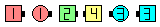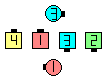Touch By 1/4 By 1/2 By 3/4\$B\$NA0(B \$B8e(BCALLERLAB definition for Touch By fraction By fraction/anythingChoreography for Touch By fraction By fraction | anythingComments? Questions? Suggestions?

https://www.ceder.net/def/touchby.php?level=C3A&language=japan&action=edit
11-December-2019 02:24:48